Mon 15 February 2021

Filed under Visualization

Tags matplotlib islr

# Minimal Visualization of Regression Results¶

## Introduction¶

I was working my way through An Introduction to Statistical Learning (https://www.statlearning.com/), and (as usual) the examples in the book were in R (to be expected from professional statisticians). My response should be "I'll learn R to replicate these!", but it is usually "How would I do this in Python?".

This post is how I replicated some graphics associated with linear regression in Python. The situation is that we have data on sales of some product in various areas, with details of money spent in advertising in three channels (TV, newspapers, and radio). We try to model the effectiveness of the three channels, based upon the sales data, and then visualize the models.

## Implementation¶

In :
%matplotlib inline

In :
%load_ext watermark

In :
%load_ext lab_black

In :
# all imports should go here

import pandas as pd
import numpy as np

import statsmodels.api as sm
from statsmodels.formula.api import ols
import statsmodels.graphics.api as smg

# housekeeping imports
import sys
import os
import subprocess
import datetime
import platform
import datetime

# graphic imports
import seaborn as sns

import matplotlib.pyplot as plt
from mpl_toolkits.mplot3d import Axes3D

# path management
from pathlib import Path


We load the data in a Pandas DataFrame, and display the first few rows.

In :
data_dir_path = Path('d:/IntroToStatLearning/')


Out:
Unnamed: 0 TV radio newspaper sales
0 1 230.1 37.8 69.2 22.1
1 2 44.5 39.3 45.1 10.4
2 3 17.2 45.9 69.3 9.3
3 4 151.5 41.3 58.5 18.5
4 5 180.8 10.8 58.4 12.9

## Analysis¶

In the analysis phase. we first try to fit a linear relationship between the individual spend in advertsing channels, and the sales. We start with the TV spend.

### TV Alone¶

We peform a Ordinary Least Squares fit of a linear relationship between TV spend, and sales, and print s asummary of the results.

In :
res1 = ols('sales ~ TV ', data=ads).fit()
res1.summary()

Out:
Dep. Variable: R-squared: sales 0.612 OLS 0.610 Least Squares 312.1 Mon, 15 Feb 2021 1.47e-42 11:41:55 -519.05 200 1042. 198 1049. 1 nonrobust
coef std err t P>|t| [0.025 0.975] 7.0326 0.458 15.360 0.000 6.130 7.935 0.0475 0.003 17.668 0.000 0.042 0.053
 Omnibus: Durbin-Watson: 0.531 1.935 0.767 0.669 -0.089 0.716 2.779 338

Warnings:
 Standard Errors assume that the covariance matrix of the errors is correctly specified.

We note that there is (almost certainly) a relationship between TV spend and sales, but with R^2 = 0.612, there is a lot of variation in the sales data left unexplained by this simple model.

### Visualization¶

Now we visualize the regression model we have just created.

We start by ploting the sales data against TV spend (in practise, we would probably do this as part of initial data exploration). We use matplotlib quick and minimal plot facilities, but will add more detail later.

In :
plt.plot(
)

Out:
[<matplotlib.lines.Line2D at 0x239dd4e4430>]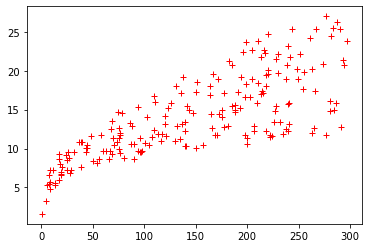We can see that the variation of the sales data is not uniform, but there is a definite upwards trend.

Now we plot the OLS line overv the range of the TV spend data. We use the Regression Results params member to get the coefficients of the model.

In :
x_range = np.asarray([min(ads['TV']), max(ads['TV'])])
plt.plot(
x_range,
res1.params['Intercept'] + res1.params['TV'] * x_range,
'g-',
)

Out:
[<matplotlib.lines.Line2D at 0x239dd58e190>]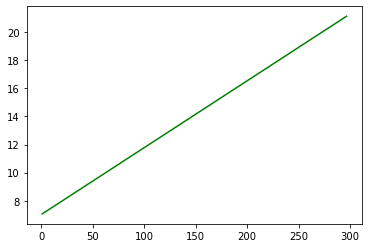Now we put these two plots together. For each data point, we draw a small red dot ('ro', ms=2), and then draw a thin (lw=1) faint (alpha=0.2) black line to the regression line. Then we draw the regression line in green, and label the X and Y axis.

In :
fig, ax = plt.subplots(figsize=(12, 8),)
for x, y_seen, y_fit in zip(
):
ax.plot(x, y_seen, 'ro', ms=2)
ax.plot([x, x], [y_seen, y_fit], 'k-', lw=1, alpha=0.2)

# end for
ax.plot(
x_range,
res1.params['Intercept'] + res1.params['TV'] * x_range,
'g-',
)
ax.set_xlabel('TV')
ax.set_ylabel('Sales')

Out:
Text(0, 0.5, 'Sales')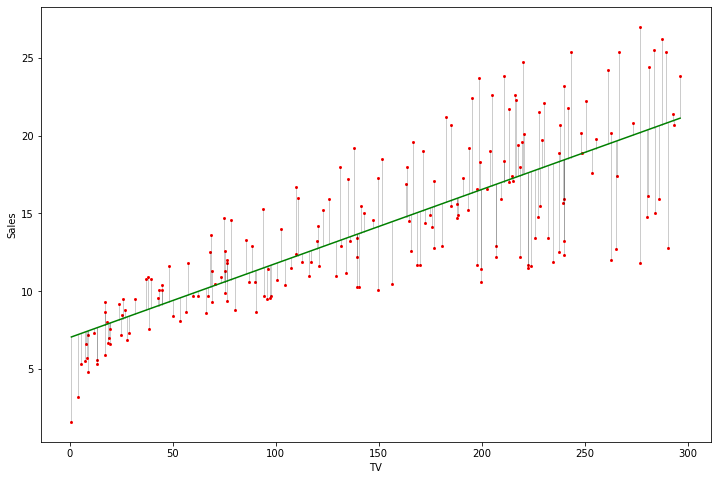There are clearly issues with the model as depicted above. The errors (actual-predicted) are not uniform, and for small values of TV spend, the error is consistently negative.

For interest, we show the mean and observation 95% Confidence Intervals (CIs) below. We get a linear spread of values of TV spend, and use the get_prediction method to get a DataFrame with CI values for these input values

We then add these Confidence Intervals to the graphic we had before.

In :
x_ci = np.linspace(0, 300, 20)
gp = res1.get_prediction({'TV': x_ci},)
pred_df = gp.summary_frame()

In :
fig, ax = plt.subplots(figsize=(12, 8),)
for x, y_seen, y_fit in zip(
):
ax.plot(x, y_seen, 'ro', ms=2)
ax.plot([x, x], [y_seen, y_fit], 'k-', lw=1, alpha=0.2)

# end for
ax.plot(
x, y_seen, 'ro', ms=2, label='Actual',
)

ax.plot(
x_range,
res1.params['Intercept'] + res1.params['TV'] * x_range,
'g-',
label='Fitted Line',
)
ax.set_xlabel('TV')
ax.set_ylabel('Sales')

gp = res1.get_prediction({'TV': x_ci})
pred_df = gp.summary_frame()
ax.plot(x_ci, pred_df['mean_ci_upper'], 'b-')
ax.plot(
x_ci, pred_df['mean_ci_lower'], 'b-', label='Mean CI',
)
ax.plot(x_ci, pred_df['obs_ci_upper'], 'b:')
ax.plot(
x_ci, pred_df['obs_ci_lower'], 'b:', label='Obs. CI',
)
ax.legend()

Out:
<matplotlib.legend.Legend at 0x239de1d6220>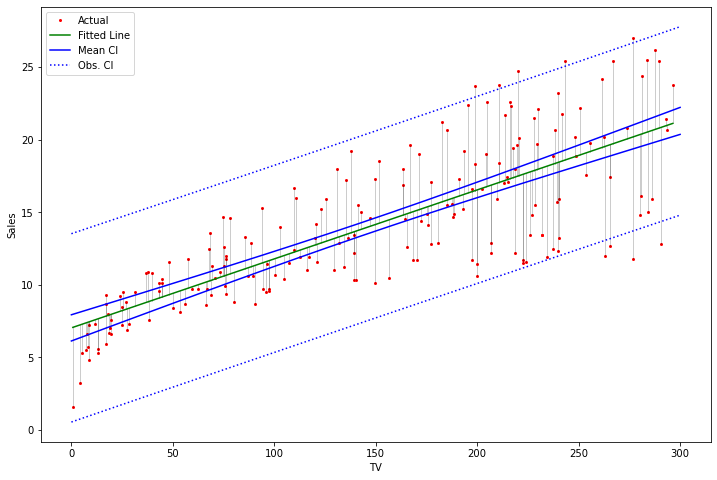The summary_frame of the predictions looks like:

In :
pred_df.head()

Out:
mean mean_se mean_ci_lower mean_ci_upper obs_ci_lower obs_ci_upper
0 7.032594 0.457843 6.129719 7.935468 0.543349 13.521838
1 7.783172 0.421674 6.951623 8.614722 1.303466 14.262878
2 8.533751 0.386792 7.770990 9.296512 2.062513 15.004988
3 9.284329 0.353577 8.587069 9.981589 2.820485 15.748174
4 10.034908 0.322544 9.398844 10.670971 3.577378 16.492437

## Further simple models¶

Next we construct individual linear models for the relationship between sales and spend on radio and newspapers. In summary, these explain little of the variance in the sales dataset (R^2 values).

In :
res1 = ols('sales ~ radio ', data=ads).fit()
res1.summary()

Out:
Dep. Variable: R-squared: sales 0.332 OLS 0.329 Least Squares 98.42 Mon, 15 Feb 2021 4.35e-19 11:41:58 -573.34 200 1151. 198 1157. 1 nonrobust
coef std err t P>|t| [0.025 0.975] 9.3116 0.563 16.542 0.000 8.202 10.422 0.2025 0.020 9.921 0.000 0.162 0.243
 Omnibus: Durbin-Watson: 19.358 1.946 0 21.91 -0.764 1.75e-05 3.544 51.4

Warnings:
 Standard Errors assume that the covariance matrix of the errors is correctly specified.
In :
res1 = ols('sales ~ newspaper ', data=ads).fit()
res1.summary()

Out:
Dep. Variable: R-squared: sales 0.052 OLS 0.047 Least Squares 10.89 Mon, 15 Feb 2021 0.00115 11:41:58 -608.34 200 1221. 198 1227. 1 nonrobust
coef std err t P>|t| [0.025 0.975] 12.3514 0.621 19.876 0.000 11.126 13.577 0.0547 0.017 3.300 0.001 0.022 0.087
 Omnibus: Durbin-Watson: 6.231 1.983 0.044 5.483 0.33 0.0645 2.527 64.7

Warnings:
 Standard Errors assume that the covariance matrix of the errors is correctly specified.

### Linear model with all variables¶

If we fit a linear model with all variables included, we get a much better explanation of the variation in the salses dataset (R^2 = 0.897). Unexpectedly, we find a weakly negative relationship between newspaper spend and sales! In fact, the 95% CI for the newspaper coefficient includes zero.

In :
res4 = ols('sales ~ TV + newspaper + radio', data=ads).fit()
res4.summary()

Out:
Dep. Variable: R-squared: sales 0.897 OLS 0.896 Least Squares 570.3 Mon, 15 Feb 2021 1.58e-96 11:41:58 -386.18 200 780.4 196 793.6 3 nonrobust
coef std err t P>|t| [0.025 0.975] 2.9389 0.312 9.422 0.000 2.324 3.554 0.0458 0.001 32.809 0.000 0.043 0.049 -0.0010 0.006 -0.177 0.860 -0.013 0.011 0.1885 0.009 21.893 0.000 0.172 0.206
 Omnibus: Durbin-Watson: 60.414 2.084 0 151.241 -1.327 1.44e-33 6.332 454

Warnings:
 Standard Errors assume that the covariance matrix of the errors is correctly specified.

We can use numpy to get the correlation between the various variables, and pandas to turn this into a DataFrame for a more polished presentation. We find that the newspaper spend is most strongly correlated with the radio spend.

In :
cm = np.corrcoef(
rowvar=False,
)
cm

Out:
array([[1.        , 0.05480866, 0.05664787, 0.78222442],
[0.05480866, 1.        , 0.35410375, 0.57622257],
[0.05664787, 0.35410375, 1.        , 0.22829903],
[0.78222442, 0.57622257, 0.22829903, 1.        ]])

We show the matrix in a more civilized format

In :
cm_df = pd.DataFrame(
data=cm,
)
cm_df

Out:
TV 1.000000 0.054809 0.056648 0.782224
newspaper 0.056648 0.354104 1.000000 0.228299
sales 0.782224 0.576223 0.228299 1.000000

### Final linear model¶

In our final purely linear model, we drop the newspaper spend (and find no reduction in the explained variation in the sales dataset).

In :
res5 = ols('sales ~ TV + radio', data=ads).fit()
res5.summary()

Out:
Dep. Variable: R-squared: sales 0.897 OLS 0.896 Least Squares 859.6 Mon, 15 Feb 2021 4.83e-98 11:41:59 -386.20 200 778.4 197 788.3 2 nonrobust
coef std err t P>|t| [0.025 0.975] 2.9211 0.294 9.919 0.000 2.340 3.502 0.0458 0.001 32.909 0.000 0.043 0.048 0.1880 0.008 23.382 0.000 0.172 0.204
 Omnibus: Durbin-Watson: 60.022 2.081 0 148.679 -1.323 5.19e-33 6.292 425

Warnings:
 Standard Errors assume that the covariance matrix of the errors is correctly specified.

The parameters of this model can be accessed via the params attribute of the regression results.

In :
res5.params

Out:
Intercept    2.921100
TV           0.045755
dtype: float64

n_points will be the number of points we use in plotting results

In :
n_points = 20


We now plot the actuals vs predicted, using the 3D features of matplotlib

In :
fig = plt.figure(figsize=(12, 8),)

# draw the raw data points
ax.scatter(
c='red',
alpha=0.5,
)

# label the X, Y, Z axis
ax.set_xlabel('TV')
ax.set_zlabel('sales')

# plot faint vertical lines from the data points to X,Y plane
ax.plot(
[x, x], [y, y], [0, z], 'b-', alpha=0.1,
)
# end for

# plot thicker red lines from each data point to predicted value for that data point
for x, y, z1, z2 in zip(
):
ax.plot(
[x, x], [y, y], [z1, z2], 'r-', alpha=0.8,
)
# end for

# get the linear span of the X and Y axis values
tv_range = np.linspace(
)
)

# get datapoints for lines in the vertical planes for X = 0, X= Max(X) and Y=0, Y=Max(Y)
line_tv = (
tv_range * res5.params['TV'] + res5.params['Intercept']
)
+ res5.params['Intercept']
)

line2_tv = (
tv_range * res5.params['TV']
+ res5.params['Intercept']
+ np.ones(n_points)
)
+ res5.params['Intercept']
+ np.ones(n_points) * max(tv_range) * res5.params['TV']
)

# plot lines in the vertical planes for X = 0, X= Max(X) and Y=0, Y=Max(Y)
ax.plot(tv_range, np.zeros(n_points), line_tv, 'g-')

ax.plot(
tv_range,
line2_tv,
'g-',
)

ax.plot(
np.ones(20) * max(tv_range),
'g-',
)

# plot the predicted values as a mesh grid, and as a colored surface
Z = (
X * res5.params['TV']
+ res5.params['Intercept']
)

surf = ax.plot_wireframe(X, Y, Z, color='green', alpha=0.4)
surfs = ax.plot_surface(X, Y, Z, color='green', alpha=0.1)

# set the viewing angle
ax.view_init(elev=20, azim=60)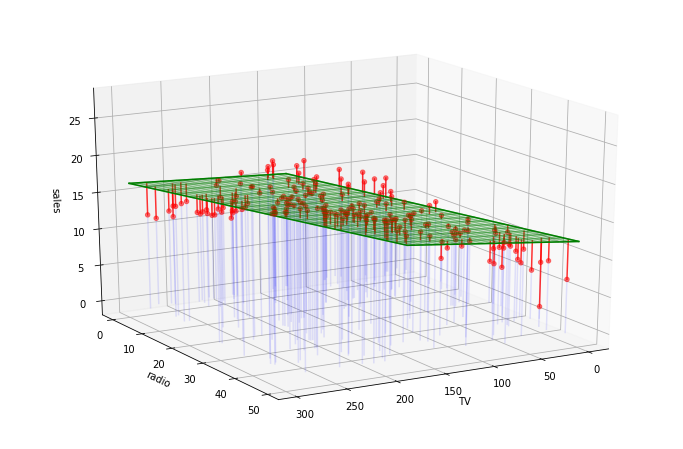We can see that the prediction errors are not distributed uniformly across the range of the datasets (e.g. the prediction errors are uniformly positive at the left and right corners of the prediction plane, as shown above (predicted > actual).

We can also plot the raw data values in the X=0, and Y=0 planes, as below.

In :
fig = plt.figure(figsize=(12, 8),)

# do 3d scatter plot
ax.scatter(
c='red',
alpha=0.5,
)

# set X,Y,Z axis labels
ax.set_xlabel('TV')
ax.set_zlabel('sales')

# draw faint line from scatter point to TV/radio plane (sales==0)
ax.plot(
[x, x], [y, y], [0, z], 'b-', alpha=0.1,
)
# end for

# show raw datapoints in radio=0 plane
ax.scatter(
c='blue',
alpha=0.3,
)

# show raw datapoints in TV=0 plane
ax.scatter(
c='blue',
alpha=0.3,
)

# draw red line from scatter point to fitted point
for x, y, z1, z2 in zip(
):
ax.plot(
[x, x], [y, y], [z1, z2], 'r-', alpha=0.8,
)
# end for

# get range of X, Y (or TV, radio) values for plotting
tv_range = np.linspace(
)
)

# get fitted line values on radio==0 plane, and tv==0 plane
line_tv = (
tv_range * res5.params['TV'] + res5.params['Intercept']
)
+ res5.params['Intercept']
)

line2_tv = (
tv_range * res5.params['TV']
+ res5.params['Intercept']
+ np.ones(n_points)
)

# get fitted line values on tv=max(tv) plane
+ res5.params['Intercept']
+ np.ones(n_points) * max(tv_range) * res5.params['TV']
)

# draw fitted lines in the tv==0 , and the radio==0  planes
ax.plot(tv_range, np.zeros(n_points), line_tv, 'g-')

# draw fitted lines in the tv== max(tv), and the radio==max(radio) planes
ax.plot(
tv_range,
line2_tv,
'g-',
)

ax.plot(
np.ones(20) * max(tv_range),
'g-',
)

Z = (
X * res5.params['TV']
+ res5.params['Intercept']
)

# draw wireframe and surface
surf = ax.plot_wireframe(X, Y, Z, color='green', alpha=0.4)
surfs = ax.plot_surface(X, Y, Z, color='green', alpha=0.1)

ax.view_init(elev=50, azim=50)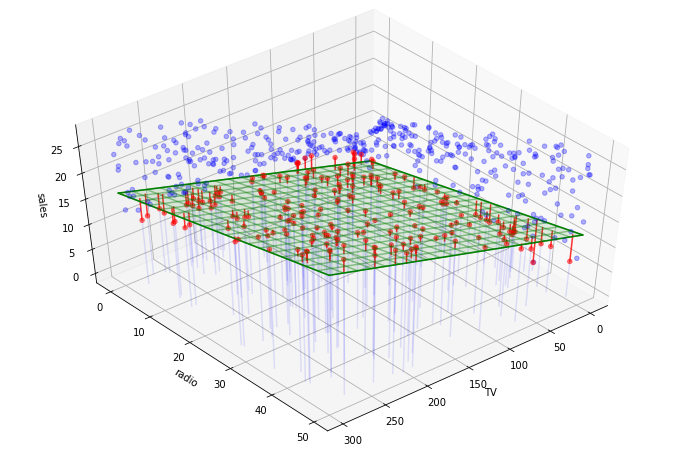## Interaction Effects¶

In our final model, we consider an interaction effect. The idea is that maybe spending on TV increases the effectiveness of radio, and vice versa. We find an interaction effect that is statistically greater than zero. R^2 has gone from 0.897 to 0.968, so almost all of the variation in the ads dataset is explained by this model.

In :
res5 = ols('sales ~ TV * radio', data=ads).fit()
res5.summary()

Out:
Dep. Variable: R-squared: sales 0.968 OLS 0.967 Least Squares 1963. Mon, 15 Feb 2021 6.68e-146 11:42:02 -270.14 200 548.3 196 561.5 3 nonrobust
coef std err t P>|t| [0.025 0.975] 6.7502 0.248 27.233 0.000 6.261 7.239 0.0191 0.002 12.699 0.000 0.016 0.022 0.0289 0.009 3.241 0.001 0.011 0.046 0.0011 5.24e-05 20.727 0.000 0.001 0.001
 Omnibus: Durbin-Watson: 128.132 2.224 0 1183.72 -2.323 9.09e-258 13.975 18000

Warnings:
 Standard Errors assume that the covariance matrix of the errors is correctly specified.
 The condition number is large, 1.8e+04. This might indicate that there are
strong multicollinearity or other numerical problems.

### Final model visualized¶

When we visualize the model, we can see that the distribution of the residuals (actual-predicted) is now much more uniform.

In :
fig = plt.figure(figsize=(12, 8),)

# do 3d scatter plot
ax.scatter(
c='red',
alpha=0.5,
)

# set X,Y,Z axis labels
ax.set_xlabel('TV')
ax.set_zlabel('sales')

# draw faint line from scatter point to TV/radio plane (sales==0)
ax.plot(
[x, x], [y, y], [0, z], 'b-', alpha=0.1,
)
# end for

# draw line from scatter point to fitted point
for x, y, z1, z2 in zip(
):
ax.plot(
[x, x], [y, y], [z1, z2], 'r-', alpha=0.8,
)
# end for

Z = (
X * res5.params['TV']
+ X * Y * res5.params['TV:radio']
+ res5.params['Intercept']
)

# draw wireframe and surface
surf = ax.plot_wireframe(X, Y, Z, color='green', alpha=0.4)
surfs = ax.plot_surface(X, Y, Z, color='green', alpha=0.1)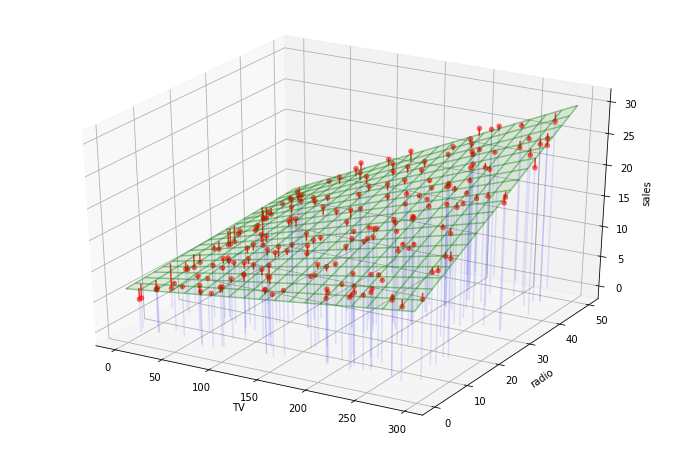## Summary¶

The comparion with the graphics in ITSL is interesting (I assume the graphics in ITSL are done with R). By default, matplotlib 3D places ticks and tick labels, and shows the 3D aspect by a grid in each of the figure 'back planes'. The ITSL graphics are very much paired back, with no ticks or tick labels, and no grids. I can achieve the same result, as below.

In :
fig = plt.figure(figsize=(12, 8),)

# clear ticks and labels
ax.set_zticks([])
ax.set_yticks([])
ax.set_xticks([])

# clear background panes
ax.xaxis.pane.fill = False
ax.yaxis.pane.fill = False
ax.zaxis.pane.fill = False

# turn off wireframe at back of 3D plot
ax.zaxis.pane.set_edgecolor('white')
ax.yaxis.pane.set_edgecolor('white')
ax.xaxis.pane.set_edgecolor('white')

# do 3d scatter plot
ax.scatter(
c='red',
alpha=0.5,
)

# set X,Y,Z axis labels
ax.set_xlabel('TV')
ax.set_zlabel('sales')

# draw faint line from scatter point to TV/radio plane (sales==0)
ax.plot(
[x, x], [y, y], [0, z], 'b-', alpha=0.1,
)
# end for

# draw line from scatter point to fitted point
for x, y, z1, z2 in zip(
):
ax.plot(
[x, x], [y, y], [z1, z2], 'r-', alpha=0.8,
)
# end for

Z = (
X * res5.params['TV']
+ X * Y * res5.params['TV:radio']
+ res5.params['Intercept']
)

# draw wireframe and surface
surf = ax.plot_wireframe(X, Y, Z, color='green', alpha=0.4)
surfs = ax.plot_surface(X, Y, Z, color='green', alpha=0.1)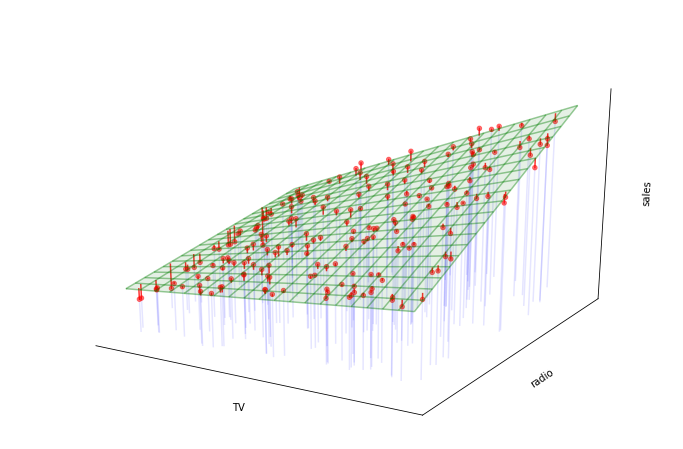## Conclusion¶

Very similar minimalist graphics can be achieved in Python, to match those shown in the ITSL book.

## Reproducibility¶

### Notebook version status¶

In :
theNotebook = 'ISLR-LinReg'

In :
# show info to support reproducibility

def python_env_name():
envs = subprocess.check_output(
'conda env list'
).splitlines()
# get unicode version of binary subprocess output
envu = [x.decode('ascii') for x in envs]
active_env = list(
filter(lambda s: '*' in str(s), envu)
)
env_name = str(active_env).split()
return env_name

# end python_env_name

print('python version : ' + sys.version)
print('python environment :', python_env_name())
print('pandas version : ' + pd.__version__)

print('current wkg dir: ' + os.getcwd())
print('Notebook name: ' + theNotebook)
print(
'Notebook run at: '
+ str(datetime.datetime.now())
+ ' local time'
)
print(
'Notebook run at: '
+ str(datetime.datetime.utcnow())
+ ' UTC'
)
print('Notebook run on: ' + platform.platform())

python version : 3.8.3 (default, Jul  2 2020, 17:30:36) [MSC v.1916 64 bit (AMD64)]
python environment : renviron
pandas version : 1.0.5
current wkg dir: C:\Users\donrc\Documents\JupyterNotebooks\IntroToStatsLearningNotebookProject\develop
Notebook name: ISLR-LinReg
Notebook run at: 2021-02-15 11:42:39.012874 local time
Notebook run at: 2021-02-15 01:42:39.012874 UTC
Notebook run on: Windows-10-10.0.18362-SP0

In :
%watermark

2021-02-15T11:42:39+10:00

CPython 3.8.3
IPython 7.16.1

compiler   : MSC v.1916 64 bit (AMD64)
system     : Windows
release    : 10
machine    : AMD64
processor  : Intel64 Family 6 Model 94 Stepping 3, GenuineIntel
CPU cores  : 8
interpreter: 64bit

In :
%watermark -h -iv

numpy           1.18.5
platform        1.0.8
statsmodels.api 0.11.1
pandas          1.0.5
seaborn         0.11.0
host name: DESKTOP-SODFUN6

In :
import matplotlib

matplotlib.__version__

Out:
'3.2.2'

Wed 21 October 2020

Filed under Geopandas

Drawing a Choropleth in an Equal Area Projection

Thu 27 August 2020

Filed under Cloud

Tags cloud aws

Third part of Cloud Resume Challenge - AWS SAM

Mon 17 August 2020

Filed under Cloud

Tags cloud aws

Second part of Cloud Resume Challenge